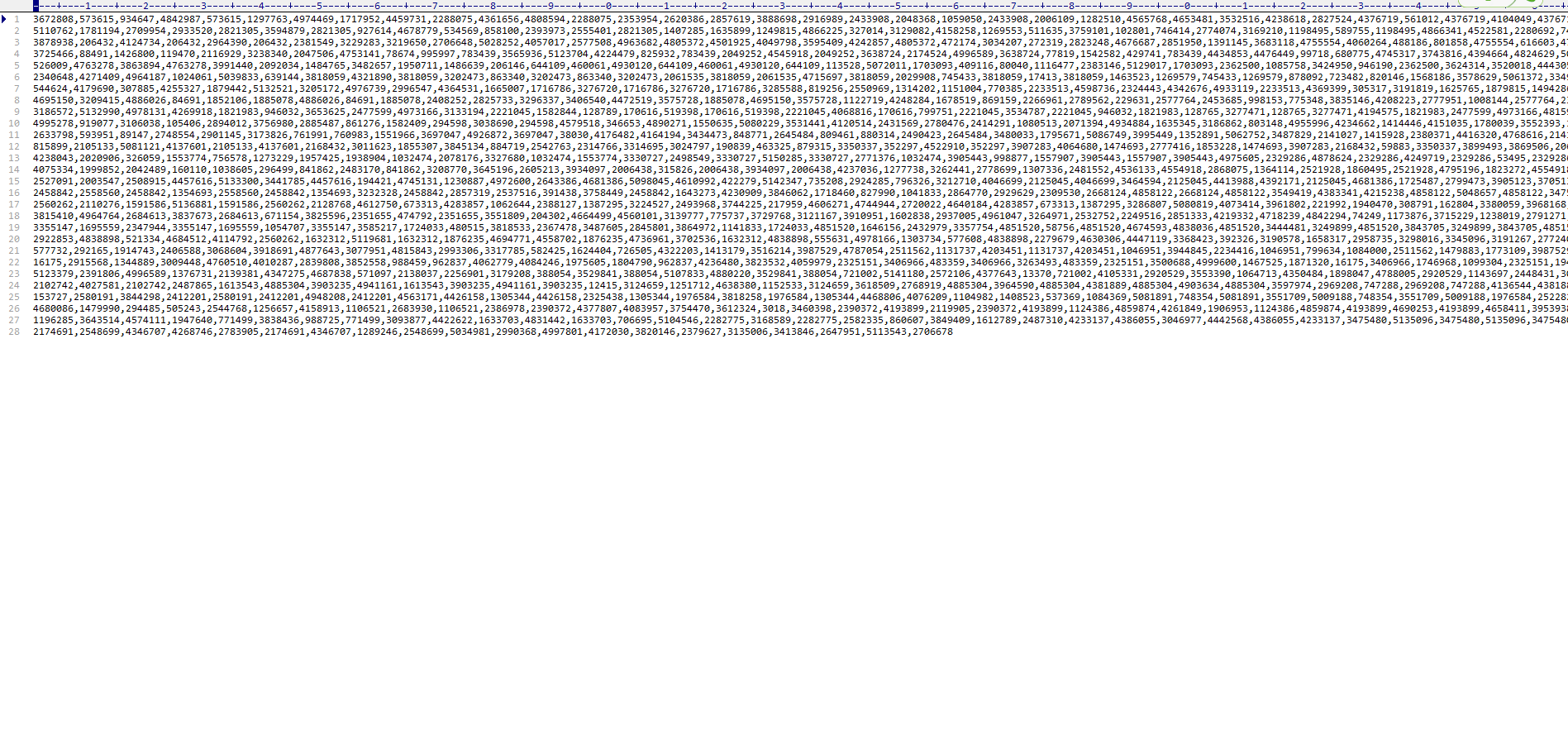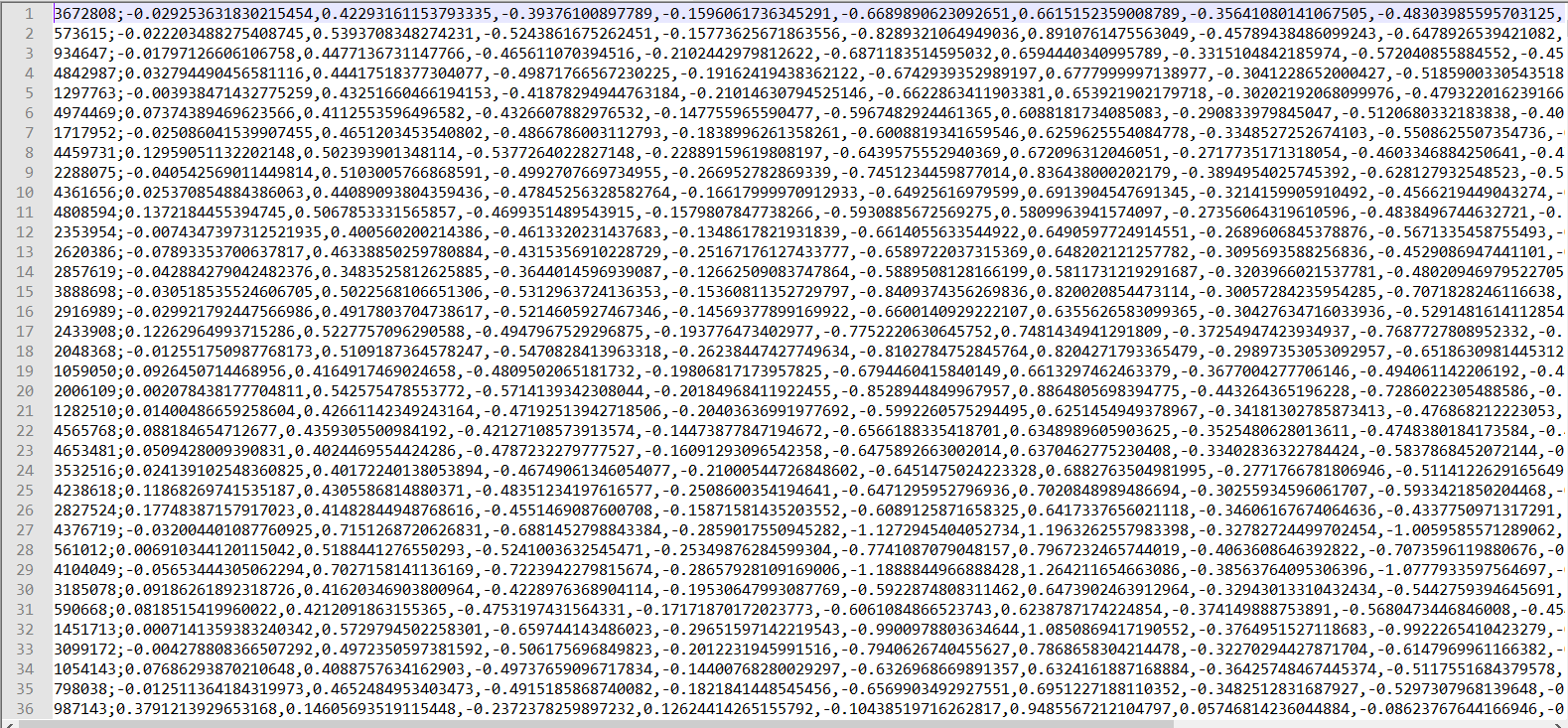• 推荐算法中的word2vec大牛直接用的该库源码，然后这个玩意目前我无法得到，所以有看到word2vec库，然而这个库不能直接在win下用pip安装，所以我先试试在服务器能不能行？ 服务器是可以的，依赖环境Cython。不过有个...
• Item2vec_Tutorial 本教程将带您了解非自然语言处理领域中Word2Vec及其扩展之一-Item2Vec背后的思想。 具体来说，使用Word2Vec的概念和Gensim软件包提供的模块，我们将构建一个轻量级的电影推荐系统。 诸如Word2...
• ## item2vec代码

千次阅读 2019-08-22 09:07:56
item2vec = SGNS(len(item), 10) # ~ print(item2vec) optimizer = optim.Adam(item2vec.parameters(), lr=0.001) for epoch in range(1): for i, batch in enumerate(get_batch_sample(100, train_data)):...
#-*-coding:utf-8-*-
"""
@author:taoshouzheng
@time:2019/8/20 10:46
@email:tsz1216@sina.com
"""

import torch
import torch.nn as nn
import torch.optim as optim
import random
from collections import Counter

class SGNS(nn.Module):

def __init__(self, vocab_size, projection_dim):

super(SGNS, self).__init__()

self.embedding_v = nn.Embedding(vocab_size, projection_dim)		# center embedding
self.embedding_u = nn.Embedding(vocab_size, projection_dim)		# out embedding
self.log_sigmoid = nn.LogSigmoid()

init_range = (2.0 / (vocab_size + projection_dim)) ** 0.5		# Xavier init
self.embedding_v.weight.data.uniform_(-init_range, init_range)		# init
# self.embedding_u.weight.data.uniform_(-0.0, 0.0)		# init
self.embedding_u.weight.data.uniform_(-init_range, init_range)  # init

def forward(self, center_words, target_words, negative_words):
center_embeds = self.embedding_v(center_words)		# B * 1 * D
target_embeds = self.embedding_u(target_words)		# B * 1 * D
neg_embeds = -self.embedding_u(negative_words)		# B * K * D
positive_score = target_embeds.bmm(center_embeds.transpose(1, 2)).squeeze(2)		# B * 1
negative_score = torch.sum(neg_embeds.bmm(center_embeds.transpose(1, 2)).squeeze(2), 1).view(center_words.size(0), -1)		# B * K ---> B * 1
los = self.log_sigmoid(positive_score) + self.log_sigmoid(negative_score)

return -torch.mean(los)

# 获取单词的embedding
def prediction(self, inputs):
embeds = self.embedding_v(inputs)
return embeds

def get_batch_sample(bat_size, tra_data):		# ~
random.shuffle(tra_data)		# 随机打乱数据
s_index = 0
e_index = bat_size

while e_index < len(tra_data):
bat = tra_data[s_index: e_index]
temp = e_index
e_index = e_index + bat_size
s_index = temp
yield bat

if e_index >= len(tra_data):
bat = train_data[s_index:]
yield bat

# 生成正样本
def get_positive_sample(samp_lists):		# ~
"""
:param :list: 二维列表
:return:
"""

positive_samples = []

for sublist in samp_lists:

sublist_length = len(sublist)

for ite in sublist:

ite_index = sublist.index(ite)

for j in range(sublist_length):

if ite_index != j:
positive_samples.append([ite, sublist[j]])

target_words = []
context_words = []

for word_pair in positive_samples:
target_words.append(word_pair)
context_words.append(word_pair)

return target_words, context_words		# 一维列表

# 生成负样本
def get_negative_sample(centers, targets, un_table, dict, k):

batch_size = len(targets)		# 批次大小

negative_samples = []

for i in range(batch_size):

neg_sample = []
center_index = centers[i]		# !!!
target_index = targets[i]		# !!!

while len(neg_sample) < k:

neg = random.choice(un_table)
if neg == target_index or neg == center_index:
continue
neg_sample.append(dict[neg])
negative_samples.append(neg_sample)

# 返回一个二维列表
return negative_samples

if __name__ == '__main__':

movie_lists = []

with open(r'E:\Experiment\Algorithms\Item2vec-pytorch\1.csv', 'r', encoding='utf8') as f:
for content in contents:
content = content.strip().split(',')
if content == '':
continue
movie_list = [int(m) for m in content]
if len(movie_list) > 1:
movie_lists.append(movie_list)		# 二维列表

fla = lambda k: [i for sublist in k for i in sublist]
item_counter = Counter(fla(movie_lists))
item = [w for w, c in item_counter.items()]  # item列表

item2index = {}
for vo in item:
if item2index.get(vo) is None:
item2index[vo] = len(item2index)

index2item = {v: k for k, v in item2index.items()}

new_movie_lists = []
for m in movie_lists:
m = [item2index[n] for n in m]
new_movie_lists.append(m)

cent_words, cont_words = get_positive_sample(new_movie_lists)  # 一维列表

uni_table = []
f = sum([item_counter[it] ** 0.75 for it in item])		# 这边有个地方索引没转过来
z = 0.0001
for it in item:
uni_table.extend([it] * int(((item_counter[it] ** 0.75) / f) / z))

train_data = [[cent_words[i], cont_words[i]] for i in range(len(cent_words))]		# 二维列表

item2vec = SGNS(len(item), 10)		# ~
print(item2vec)

for epoch in range(1):

for i, batch in enumerate(get_batch_sample(100, train_data)):

target = [[p] for p in batch]
context = [[q] for q in batch]
negative = get_negative_sample(centers=target, targets=context, un_table=uni_table, dict=item2index, k=10)

target = Variable(torch.LongTensor(target))
# print(target)
context = Variable(torch.LongTensor(context))
# print(context)
negative = Variable(torch.LongTensor(negative))
# print(negative)

loss = item2vec(target, context, negative)

loss.backward()
optimizer.step()

print('Epoch : %d, Batch : %d, loss : %.04f' % (epoch + 1, i + 1, loss))

item2vec.eval()

print(item2vec.prediction(torch.LongTensor()))
print(item2vec.prediction(torch.LongTensor()).data.numpy().tolist())
print(item2vec.prediction(torch.LongTensor()).data.size())

item_embeddings = []

for item, index in item2index.items():

print(item)
print(index)
print(torch.flatten(item2vec.prediction(torch.LongTensor([index]))).data)

item_embedding_str = str(item) + ';' + ','.join([str(a) for a in torch.flatten(item2vec.prediction(torch.LongTensor([index]))).data.numpy().tolist()])

item_embeddings.append(item_embedding_str)

with open(r'E:\Experiment\Algorithms\Item2vec-pytorch\em.txt', 'w', encoding='utf8') as g:
for st in item_embeddings:
g.write(st + '\n')

print('完成！')

输入数据如下：结果如下：展开全文• ## item2vec详解

千次阅读 2020-04-07 23:04:13
1.item2vec的兴起 自从word2vec被证明效果不错以后，掀起了一股embedding的热潮。item2vec, doc2vec，总结起来就是everything2vec。在实际工作中，embedding的使用也非常广泛，今天我们就来说说常用的item2vec。 ...
项目github地址：bitcarmanlee easy-algorithm-interview-and-practice 欢迎大家star，留言，一起学习进步
1.item2vec的兴起
自从word2vec被证明效果不错以后，掀起了一股embedding的热潮。item2vec, doc2vec，总结起来就是everything2vec。在实际工作中，embedding的使用也非常广泛，今天我们就来说说常用的item2vec。
word2vec构建样本时，句子分词以后就构成了一个天然的序列。那么在推荐的应用场景下，如何生成这个序列，或者说如何生成训练样本，成为了一个必须要解决的问题。
一般来说，有如下两种方式构建训练样本 1.基于时序的关系 我们会认为item之间存在时序关系，相当于我们将各个不同的item类比为word2vec的单词，那么将这些item串联起来就相当于将word连起来组成一个句子，这样不管是构造训练集的方式或者训练的方式都可以按照word2vec的方式来进行了。 比如视频网站的用户观看的视频序列，音乐网站用户听的歌曲序列，我们都可以认为是这种方式。
2.基于集合的关系 认为 item 之间存在非常弱的时序关系，或者因为某种原因，我们无法获得 item 的序列。那么这种情况只能放弃考虑 item 间的时空信息，转而使用 item 集合来取代序列。通常我们把用户的行为序列视为一个集合（当然还有其他集合构造方式），我们假定一个静态的环境，在这个环境中，共现在同一个集合的 item 就可以认为它们是相似的，然后视为正样本，不管它们是以什么样的顺序产生的，如果某两个 item 频繁共现，二者的 Embedding 则可能也很相似。很明显，这种利用集合共现性构建样本的方式与 Word2Vec 的输入样本还是不一样的，因此无法直接应用 Word2Vec 的算法，这就需要对 Word2Vec 的过程进行调整。另外还需要注意的是，上面的这种的假设是有局限性的，不一定适用于其它场景。 基于集合的示例比如电商网站订单中的商品集合， 另外比如用户的浏览物料item的集合。
2.常用的Embedding方式
1.以SVD为代表的MF方式。通过对user-item-score矩阵的分解，或者user-item的隐式矩阵分解，可以获取user与item的隐向量，该向量可以作为embedding向量使用。 2.FM算法。FM学习了各个特征的隐向量表示，从而可以将这些学习到的隐向量作为特征的embedding使用。 3.DNN-Embedding 通过神经网络，利用接入Embedding层与目标loss进行joint train，从而学习其特征表达，这是一种端到端的embedding训练方式(end-to-end)。 4.item2vec 与word2vec类似的方式。 5.graph embedding，是基于图模型的方法，包括deep walk, node2vec, eges等方法。
3.item2vec与MF的区别
首先，二者都应用了隐向量来表征实体特征，不同的是，传统的 MF 通常是 user-item 矩阵，而 Item2Vec 通过滑动窗口样本生成的方式构造出的则更像是 item-item 矩阵；另外，二者得到隐向量的方式也不同，MF 利用均方差损失，使预测得分与已有得分之间的误差尽可能地小，而 Item2Vec 则是利用空间信息并借助了最大似然估计的思想，使用对数损失，使上下文关系或者共现关系构造出的正样本的 item Pair 出现的概率可能地大；此外训练 Item2Vec 的时候还要引入负样本，这也是与 MF 不同的地方。
对于二者在推荐效果上的差异，一个经验是传统 MF 推荐会让热门内容经常性排在前面，而 Item2vec 能更好的学到中频内容的相似性。Iterm2Vec 加上较短的时间窗口，相似推荐会比 MF 好很多。
4.原理
对于出现在同一个集合的item对我们视为正样本，对于集合

ω

1

,

ω

2

,

⋯

,

ω

K

\omega_1, \omega_2, \cdots, \omega_K

，目标函数为

1

K

∑

i

=

1

K

∑

j

≠

i

K

log

⁡

p

(

w

j

∣

w

i

)

\frac{1}{K} \sum_{i=1}^{K}\sum_{j \neq i}^{K} \log p(w_j | w_i)

利用负采样，将

p

(

w

j

∣

w

i

)

p(w_j | w_i)

可以定义为

p

(

w

j

∣

w

i

)

=

e

x

p

(

u

i

T

v

j

)

∑

k

∈

I

W

e

x

p

(

u

k

T

v

j

)

p(w_j | w_i) = \frac{exp(u_i ^T v_j)}{\sum_{k \in I_W} exp(u_k ^T v_j)}

简单而言，对于给定的item序列，选择窗口大小为c，skip-gram通过当前item来预测前后c个item的概率，从而使得其后验概率最大，通过极大似然进行优化整体损失。
负采样的词频计算方式跟word2vec一样。
参考文献
1.https://lumingdong.cn/application-practice-of-embedding-in-recommendation-system.html
展开全文滑动窗口
• 微软研究人员发表的一篇关于将word2vec模型应用到协同过滤中的Items集合中，来计算items的向量化表示的论文。
• item2vecitem2vec 主流程： 从log中抽取用户行为序列 将行为序列当成预料训练word2Vec得到item embedding：把用户浏览的商品集合等价于word2vec中的word的序列. 得到item sim关系用于推荐 1、首先第一步：这是...
item2vec等
item2vec
主流程：

从log中抽取用户行为序列
将行为序列当成预料训练word2Vec得到item embedding：把用户浏览的商品集合等价于word2vec中的word的序列.
得到item sim关系用于推荐

1、首先第一步：这是我们从推荐系统log中获得的，也就是说User A行为过item a、item b、item d，User B行为过item a、item c，User C行为过item b、item e。

2、继而我们需要将这些转化成句子。句子1就是a、b、d。句子2就是a、c。句子3就是b、e。这里我们已经没有了user之间的关系，只留下item组成的句子。

3、将句子放入到放入word2vec模型，这里就是一个输入（word2vec模型是一个三层的神经网络：输入层、隐含层、输出层）

经过word2vec模型的训练，会得到每一个item对应的embedding层向量。这里只写了item a [0.1,0.2,0.14…0.3] ,item b [0.4,0.6,0.14…0.3]。item c，item d,item e也是可以得到的。

4、得到item向量之后，就得到item的sim关系。基于item sim的关系，我们便完成了用户的推荐。

（ 出现在同一个集合的商品被视为positive

word2vec 几大步骤
读取查看、点击、播放等行为数据，我用的是播放数据；
数据整理成(userid, itemid, playcnt)的形式，这个数据可能是聚合N天得到的；
过滤掉playcnt为小于3的数据，我把这些过滤掉，觉得这个数据没有贡献；
按照userid聚合，得到(userid, list(itemid))的形式；
训练word2vec；
导出model.vectors()，里面包括word和对应的向量vector，其中word其实就是itemid
crossjoin计算两两相似度，取相似度TOP N；
将结果存入mysql，后续可以加载到REDIS实现实时相似推荐；

对比ItemCF，Item2vec有更好的泛化能力，我们可以基于训练出来的item Embedding，召回邻近topK items，这突破了ItemCF邻域关系的限制。
Item2vec
主要做法是把item视为word，用户的行为序列视为一个集合，item间的共现为正样本，并按照item的频率分布进行负样本采样。缺点是相似度的计算还只是利用到了item共现信息，（1）忽略了user行为序列信息；（2）没有建模用户对不同item的喜欢程度高低。无法解决覆盖率问题，在合理阈值下，只能覆盖出现在用户行为序列中的物品集，但对于低频物品和新物品，还是没有召回结果的。虽然覆盖率略高于ItemCF，但点击率低于ItemCF，整体收益仍是负向的，位置比较尴尬。
item2item
很多场景下item2item的推荐方式要优于user2item；
item2item的推荐方式：在获取item相似度矩阵之后，根据用户的最近的行为，根据行为过的item找到相似的item，完成推荐，如itemCF。
user2item：根据用户的基本属性和历史行为等基于一定的模型，算出最可能喜欢的item列表写在KV存储中；当用户访问系统的时候，将这些item列表推荐给用户，像userCF、LFM、personal rank算法等都是这种方式。
user2vec
给定一个用户，找到联合表征向量空间中的前K个相近的产品。
训练数据集分为用户购买顺序S，即给定用户un，其按照时间来排序的已经购买的产品。un = (pn1, pn2, . . . pnUn)，Un表示用户un的项目购买数量。

怎么训练和生成user的Embedding？
item和user的量可以认为是无限的，所以不能直接使用它们的index来构建。我们可以用其某些有限的属性来表达它们。比如，说user，其实聊的是user喜欢什么item，接触过什么item，那么其中的核心其实还是item，这样理解的话，user的Embedding其实就源自于若干个与之相关的item的Embedding。
这里的核心就在于，如何结合用户有过行为的若干个item的Embedding，合成一个User Embedding。
最粗暴的方法是采用item-embedding进行平均，而序列的长度很关键，太短了无法完全表征，太长了无法可能用户兴趣发生了偏移效果不好，因而可以作为baseline(大多数情况下，效果说的过去)在上面的加上一点点改进，对应不同的推荐商品召回的情况下，采用不同的embedding，或者加上attention-layer，相当于用户的兴趣是多峰的，每次只有单峰激活(参考DIN)加上序列和时间考量，用LSTM(GRU)等处理这个“sentence”，最终最终得到的编码可以是user-embedding(DIEN)

生成item vector之后，可以根据用户历史上与item的交互行为，求avg/max/ pooling获取用户的向量表达。这是最直接的user vector生成方式，但是在实际使用中往往效果一般。主要原因是泛化能力差，对user embedding的学习是无监督的。

User Embedding的方法非常多，取决与你想怎么用，这里介绍几种工业界的方法。召回侧：
1.用户行为序列的item embedding求平均，这样求出的user embedding和item embedding是在同一个空间里的，可以直接通过user embedding和item embedding的内积形式做在线knn召回
2.进一步考虑到用户兴趣随时间的变化，把平均改为时间衰减的加权平均
3.更粗暴一些，直接用用户最近点击的那个item emebdding做user embedding，在某些业务场景下也有很好的效果

几种DNN的方式构建user embedding。
FM
介绍：https://juejin.im/post/6844904078619738126
，只需要将User Embedding和video Embedding存储到线上内存数据库，通过内积运算再排序的方法就可以得到video的排名，这大大加快了召回效率。
使用特征：用户观看过视频的 embedding 向量、用户搜索词的 embedding 向量、用户画像特征、context 上下文特征等。

训练方式：三层 ReLU 神经网络之后接 softmax 层，去预测用户下一个感兴趣的视频，输出是在所有候选视频集合上的概率分布。训练完成之后，最后一层 Relu 的输出作为 user embedding，softmax 的权重可当做当前预测 item 的 embedding 表示。

线上预测：通过 userId 找到相应的 user embedding，然后使用 KNN 方法 ( 比如 faiss ) 找到相似度最高的 top-N 条候选结果返回。


在召回DNN中，引入丰富的用户侧特征（包括用户的观看和搜索历史），模型的最后一层全连接的输出作为user embedding表达，item embedding则使用softmax+negative sampling有监督训练该DNN时，生成的softmax权重向量来表示。
DSSM
DSSM及微软后续推出的multi-view DSSM也代表了一种思路。这种多塔的结构，可以为user，item构造不同的塔，通过n层全连接之后，将最后一层输出层的向量拼接在一起做softmax，或者直接通过cosine衡量两者的相似性。训练完成后，模型全连接层的输出向量可以作为user，item的embedding表达，而且向量处在同一个向量空间，可以在线进行ANN查找，有效提高线上serving的性能。
总结
user embedding就是网络的最后一个隐层，video embedding是softmax的权重.
将最后softmax层的输出矩阵的列向量当作item embedding vector，而将softmax之前一层的值当作user embedding vector。

链接
Spark使用word2vec训练item2vec实现内容相关推
https://www.crazyant.net/2447.html
改进：
Topic2vec：https://lxmly.github.io/2019/02/15/embedding/
w-item2vec：https://www.cnblogs.com/simplekinght/p/11545180.html
https://www.infoq.cn/article/0ueIPm2VFrOqECLU3316 （深度学习技术在美图个性化推荐的应用实践）
其他代码
MF的embdding
def build_model(user_indices, item_indices, rank, ratings, user_cnt, item_cnt, lr, lamb, mu, init_value):

W_user = tf.Variable(tf.truncated_normal([user_cnt, rank], stddev=init_value/math.sqrt(float(rank)), mean=0),
name = 'user_embedding', dtype=tf.float32)
W_item = tf.Variable(tf.truncated_normal([item_cnt, rank], stddev=init_value/math.sqrt(float(rank)), mean=0),
name = 'item_embedding', dtype=tf.float32)

W_user_bias = tf.concat([W_user, tf.ones((user_cnt,1), dtype=tf.float32)], 1, name='user_embedding_bias')
W_item_bias = tf.concat([tf.ones((item_cnt,1), dtype=tf.float32), W_item], 1, name='item_embedding_bias')

user_feature = tf.nn.embedding_lookup(W_user_bias, user_indices, name = 'user_feature')
item_feature = tf.nn.embedding_lookup(W_item_bias, item_indices, name = 'item_feature')

preds = tf.add(tf.reduce_sum( tf.multiply(user_feature , item_feature) , 1), mu)

square_error = tf.sqrt(tf.reduce_mean( tf.squared_difference(preds, ratings)))
loss = square_error + lamb*(tf.reduce_mean(tf.nn.l2_loss(W_user)) + tf.reduce_mean(tf.nn.l2_loss(W_item)))

tf.summary.scalar('square_error', square_error)
tf.summary.scalar('loss', loss)
merged_summary = tf.summary.merge_all()
#tf.global_variables_initializer()

return train_step, square_error, loss, merged_summary


展开全文• ## 【推荐系统】item2vec

千次阅读 热门讨论 2019-03-14 20:16:39
Item2Vec: Neural Item Embedding for Collaborative Filtering，2016 abstract 该工作借鉴了word2vec的思想，学习item在低维隐含空间的嵌入表示，类似item-based CF用于推荐。item的序列等价于词的序列，将训练...
Item2Vec: Neural Item Embedding for Collaborative Filtering，2016
abstract

该工作借鉴了word2vec的思想，学习item在低维隐含空间的嵌入表示，类似item-based CF用于推荐。item的序列等价于词的序列，将训练样本由句子该为item序列即可，item间共现为正样本，按照item的概率分布进行负采样。实验结果表明比SVD的效果更好。

模型
skip-gram：最小化目标函数

1

K

∑

i

=

1

K

∑

−

c

≤

j

≤

c

,

j

≠

0

l

o

g

p

(

w

i

+

j

∣

w

i

)

\frac{1}{K} \sum_{i=1}^{K} \sum_{-c\leq j \leq c , j\neq0}{{\rm log \ } p(w_{i+j} | w_i)}

子采样
为了解决热门物品和冷门物品之间的不平衡，引入子采样 (subsampling)，对于输入的序列，一定概率丢弃词w

p

(

d

i

s

c

a

r

d

∣

w

)

=

1

−

ρ

f

(

w

)

其中

f

(

w

)

f(w)

为w的频率，

ρ

\rho

是预设的阈值。在本文两个数据集中分别取

1

0

−

5

10^{-5}

和

1

0

−

3

10^{-3}

。
负采样
为了加快训练，引入负采样（negtive sampling）：

p

(

w

j

∣

w

i

)

=

σ

(

u

i

T

v

j

)

∏

k

=

1

N

σ

(

−

u

i

T

v

k

)

p(w_j|w_i) = \sigma(u_i^Tv_j) \prod_{k=1}^N \sigma(-u_i^Tv_k)

σ

(

x

)

=

1

1

+

e

x

p

(

−

x

)

\sigma(x) = \frac{1}{1+exp(-x)}

，N是负样本数目
在item2vec中，将序列当作集合来处理，只要共现就视为正样本。优化的目标函数替代为

1

K

∑

i

=

1

K

∑

j

≠

i

K

l

o

g

p

(

w

j

∣

w

i

)

\frac{1}{K} \sum_{i=1}^{K} \sum_{j\neq i}^{K}{{\rm log \ } p(w_{j} | w_i)}

具体的处理方法有以下2种
（1）将窗口设置足够大，同个序列中的数据都视为正样本
（2）每次训练时打乱原序列
最终将训练好的target representation

u

i

u_i

作为最终

i

t

e

m

i

item_i

的表示
代码实现：https://github.com/zhengjingwei/recommender-system
讨论
在实际应用中item2vec的得到的embedding聚类效果好。
在推荐时，直接计算embedding的相似度取TopN作为推荐结果容易得到过于相似的商品（如同款商品的不同型号）。
代码实现
以下是参考tensorflow中word2vec basic代码改的
from __future__ import absolute_import
from __future__ import division
from __future__ import print_function

import tensorflow as tf
import numpy as np
import math
import collections
import random
from six.moves import xrange

local_file = '../data/trans_all_incode.csv' # 每行一个订单
log_dir = './log'

读数据
def read_data(filename):
corpus = []
vocabulary = set()
words = []
max_len = 0
with open(filename,'r') as f:
for line in f:
sentence =  line.strip().split('|')
corpus.append(sentence)
max_len = max(max_len,len(sentence))
words += sentence
print('corpus size: %s sentence %s words' %(len(corpus), len(words)))
print('sentence max len: %s' %(max_len))
return corpus,words

def generate_batch_from_sentence(sentence, num_skips, skip_window):
batch_inputs = []
batch_labels = []
for i in range(len(sentence)):
window = list(range(len(sentence))) # 句子内除该元素外所有元素
window.remove(i)
sample_index = random.sample(window,min(num_skips,len(window)))
input_id = word2id.get(sentence[i])
for index in sample_index:
label_id = word2id.get(sentence[index])
batch_inputs.append(input_id)
batch_labels.append(label_id)

batch_inputs = np.array(batch_inputs,dtype=np.int32)
batch_labels = np.array(batch_labels,dtype=np.int32)
batch_labels = np.reshape(batch_labels,[batch_labels.__len__(),1])
return batch_inputs,batch_labels

建立item到id以及id到item的映射
corpus,words = read_data(local_file)
vocabulary = collections.Counter(words)
count = []
count.extend(collections.Counter(words).most_common(len(words) - 1))
word2id = dict()
for word , _ in count:
word2id[word] = len(word2id)

id2word = dict(zip(word2id.values(), word2id.keys()))

模型设置
embedding_size = 100 # embedding维度
skip_window = 50     # 单边窗口长度
num_skips = 4        # 从整个窗口中选取多少个不同的词作为我们的output word
num_sampled = 50     # 负采样样本数

valid_size = 16      # 选取验证的样本数
valid_window = 500   # 在前100个的中挑选验证样本
valid_examples = np.random.choice(valid_window, valid_size, replace=False)

vocabulary_size = len(vocabulary)
batch_size = None

graph = tf.Graph()
with graph.as_default():
# Input data.
with tf.name_scope('inputs'):
train_inputs = tf.placeholder(tf.int32, shape=[batch_size])
train_labels = tf.placeholder(tf.int32, shape=[batch_size, 1])
valid_dataset = tf.constant(valid_examples, dtype=tf.int32)

# 查找输入的embedding
with tf.name_scope('embeddings'):
embeddings = tf.Variable(tf.random_uniform([vocabulary_size, embedding_size], -1.0, 1.0)) # 随机均匀分布
embed = tf.nn.embedding_lookup(embeddings, train_inputs)

# 模型内部参数矩阵初始化
with tf.name_scope('weights'):
nce_weights = tf.Variable(
tf.truncated_normal([vocabulary_size, embedding_size],
stddev=1.0 / math.sqrt(embedding_size)))

with tf.name_scope('biases'):
nce_biases = tf.Variable(tf.zeros([vocabulary_size]))

# 得到NCE损失
with tf.name_scope('loss'):
loss = tf.reduce_mean(tf.nn.nce_loss(
weights = nce_weights,
biases = nce_biases,
labels = train_labels,
inputs = embed,
num_sampled = num_sampled,
num_classes = vocabulary_size))

tf.summary.scalar('loss', loss)

with tf.name_scope('optimizer'):

# 计算与指定若干单词的相似度
norm = tf.sqrt(tf.reduce_sum(tf.square(embeddings), 1 ,keep_dims=True))
normalized_embedding = embeddings / norm
valid_embeddings = tf.nn.embedding_lookup(normalized_embedding, valid_dataset)

similarity = tf.matmul(valid_embeddings,  normalized_embedding, transpose_b=True)

merged = tf.summary.merge_all()

# 变量初始化
init = tf.global_variables_initializer()

saver = tf.train.Saver()

训练
num_steps = 1000001
with tf.Session(graph=graph) as sess:
train_loss_records = collections.deque(maxlen=10) # 保存最近10次的误差
train_loss_k10 = 0
train_sents_num = 0

# 写入summary
writter = tf.summary.FileWriter(log_dir, sess.graph)

# 初始化全局参数
init.run()
aver_loss = 0

for step in xrange(num_steps):
sentence = corpus[step % len(corpus)] # 逐句训练
batch_inputs, batch_labels = generate_batch_from_sentence(sentence, num_skips, skip_window)

feed_dict = {train_inputs: batch_inputs, train_labels: batch_labels}

_, summary, loss_val = sess.run([optimizer, merged, loss],
feed_dict=feed_dict)
#         average_loss += loss_val
train_sents_num += 1
# 每一步将summary写入writer
train_loss_records.append(loss_val)
train_loss_k10 = np.mean(train_loss_records)

if train_sents_num % 1000 == 0:
print('{a} sentences dealed, loss: {b}'
.format(a=train_sents_num,b=train_loss_k10))

if train_sents_num % 5000 == 0:
sim = similarity.eval()
for i in xrange(valid_size):
valid_word = id2word[valid_examples[i]]
top_k = 8
nearest = (-sim[i, :]).argsort()[1:top_k + 1]
log_str =  'Nearest to %s:' % valid_word

for k in xrange(top_k):
close_word = id2word[nearest[k]]
log_str = '%s %s,' % (log_str, close_word)
print(log_str)

final_embeddings = normalized_embedding.eval()


展开全文推荐系统 表示学习 embedding
• 推荐系统中，传统的CF算法都是利用 item2item 关系计算商品间相似性。i2i数据在业界的推荐系统中起着非常重要的作用。传统的i2i的主要计算方法分两类，memory-based和model-based。 本文主要介绍了microsoft和airbnb...深度学习 人工智能 推荐系统
• Item2vec 是基于自然语言处理模型 Word2vec 提出的，所以 Item2vec 要处理的是类似文本句子的观影序列： def processItemSequence(spark, rawSampleDataPath): # 读取 ratings 原始数据到 Spark 平台 ...
• 在上面的word2vec中，可以训练得到每个itemvec，当然也可根据每个item的vector计算他们之间的相似度，我推荐直接采用similar by vector，这样可以由query得到topk个最接近的词，如下： similar_by_vector(vector...NLP 推荐算法推荐系统
• 文章目录简介简单介绍一下word2vecitem2vec的原理 简介 本文可以算是一遍item2vector的工程化教程，其基本理论源自于论文《Item2Vec: Neural Item Embedding for Collaborative Filtering》...推荐系统
• 点击标题下「小小挖掘机」可快速关注本主题文章将会分为三部分介绍，每部分的主题为：word2vec的前奏-统计语言模型word2vec详解-风华不减其他xxx2vec论文和应用介绍后续会更...聚类 可视化 算法 深度学习 机器学习
• ## Item2Vec算法介绍

千次阅读 2019-03-01 22:46:18
最早看到Item2vec是微软研究院的这篇文章（https://arxiv.org/vc/arxiv/papers/1603/1603.04259v2.pdf），本文主要是对这篇论文做一些介绍，Item2vec主要思想是借鉴了Word2Vec，并且采用SGNS（Skip-gram + Negative ...推荐系统
• 通过数据统计可以发现，在q增大以后Item2vec的准确度开始显著领了SVD，在统计最不热门的10k的item过程中，发现Item2vec的准确度领先了SVD 10个百分点，说明Item2vec对于一些不热门的item也取得了较好的学习效果。...推荐系统 Embedding
• 输出端是与输入端的 target item 邻近的多个item，基本思想是用户输入某个商品后，使用embedding后的物品为输入向量，从而训练出一个向量空间，在此空间中，类似的物品的值相近，item2vec可以从已经训练好的模型...深度学习
• https://www.jianshu.com/p/0ecc53bba169推荐系统入门实践（4）召回之item2vec
• ## item2vec的理解

千次阅读 2017-10-07 11:05:30
最早提出 item2vec 这个方法是在这篇论文中Item2Vec: Neural Item Embedding for Collaborative Filtering 这里的 item2vec 用到的方法和 word2vec 中的方法基本类似。可以说是把 word2vec 中的方法迁移到了推荐...
• 用spark的机器学习库怼出一个推荐系统(二)—Word2Vec 个人观点，为了适应潮流，spark后续为一直维护它的机器学习库，目前提供的模型自然不多，本人从事的是推荐方向，推荐方向的话，工业界肯定是要排序+召回的，...scala spark 推荐系统
• total_words=max_vocabulary_size, epochs=500, start_alpha=0.1, end_alpha=0.02, compute_loss=True) model.save("gensim/item2vec.model") model.wv.save_word2vec_format("gensim/item2vec.txt", total_vec=max...
• 论文题目：《Item2Vec: Neural Item Embedding for Collaborative Filtering》 发表时间：RecSys 2016 作者及单位： 论文地址：http://ceur-ws.org/Vol-1688/paper-13.pdf 二、摘要 许多协作过滤（CF）...推荐系统 深度学习
• from gensim.models import Word2Vec import logging import sys reload(sys) sys.setdefaultencoding('utf8') from sklearn.model_selection import train_test_split c = [] def load_sequence(from_path): ...python
• gensim中word2vec API参数说明 api具体含义 model = Word2Vec(sentence, # sg=1, # sg=1则采用skip-gram算法 size=100, # 特征向量的维度，默认为100。大的size需要更多的训练数据但是效果会更好 window=5, #...
• 已经了解了Word2vec，怎么应用于推荐场景中，形成item2vec呢？【深度学习】word2vec（上） 在Item2Vec中，一个物品集合被视作nlp中的一个段落，物品集合的基本元素－物品等价于段落中的单词。因此在论文中，一个...
• 嵌入表示学习是当下研究热点，从word2vec,到node2vec, 到graph2vec，出现大量X2vec的算法。但如何构建向量嵌入理论指导算法设计？最近RWTH Aachen大学的计算机科学教授ACM Fellow Martin Grohe教授给了《X2vec： ...词嵌入
• ITEM2VEC：协同过滤中items的向量化表示
• 前言 自从Mikolov在他2013年的论文“Efficient Estimation of Word Representation in Vector Space”提出词向量的概念后，NLP领域仿佛一下子进入了embedding的世界，Sentence2Vec、Do...
• 本主题文章将会分为三部分介绍，每部分的主题为： ...来自于微软2016年发表在RecSys上的，因为word2vecitem2vec是在做推荐系统过程中比较常用的两个算法，所以该部分先介绍item2vec，然后再展开其他xxx2.推荐系统...